# Lines Segments and Rays What is Geometry Geometry

• Slides: 21Lines, Segments and Rays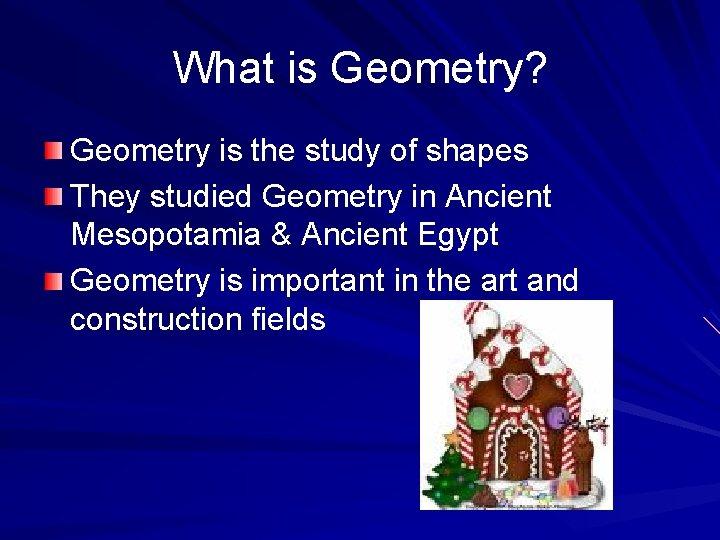What is Geometry? Geometry is the study of shapes They studied Geometry in Ancient Mesopotamia & Ancient Egypt Geometry is important in the art and construction fields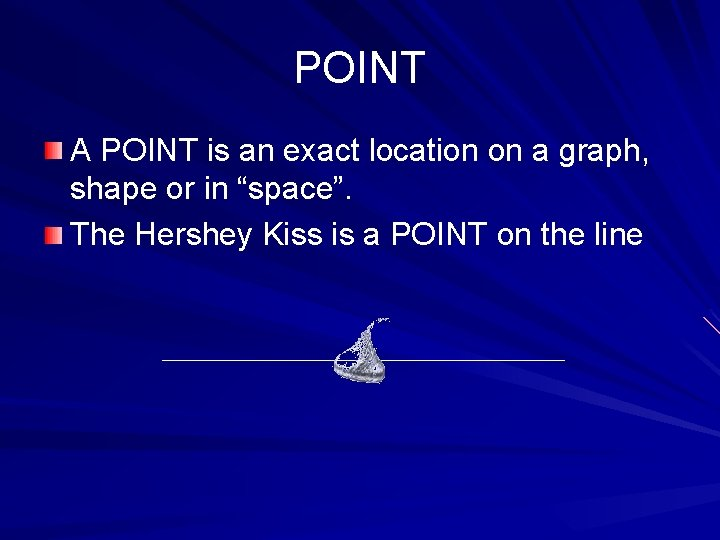POINT A POINT is an exact location on a graph, shape or in “space”. The Hershey Kiss is a POINT on the line ●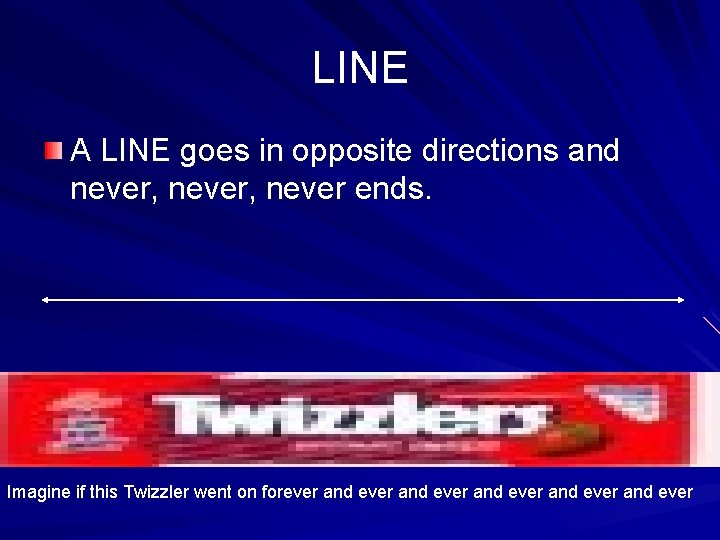LINE A LINE goes in opposite directions and never, never ends. Imagine if this Twizzler went on forever and ever and everRAY A RAY is part of a line, but it has one endpoint and the other end keeps going. ● Raaaaaaaaaaaayyyyyyyyyyyyyy EndpointENDPOINT An ENDPOINT is a point at the end of a ray or line segment. Endpoint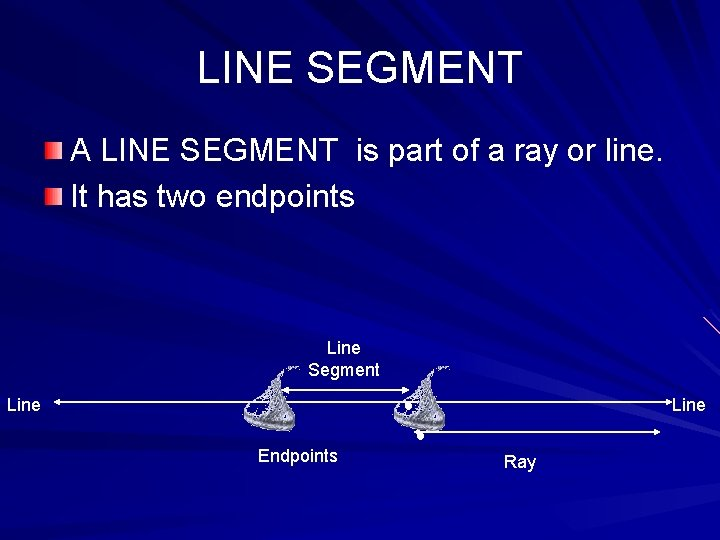LINE SEGMENT A LINE SEGMENT is part of a ray or line. It has two endpoints Line Segment Line ● ● Line ● Endpoints Ray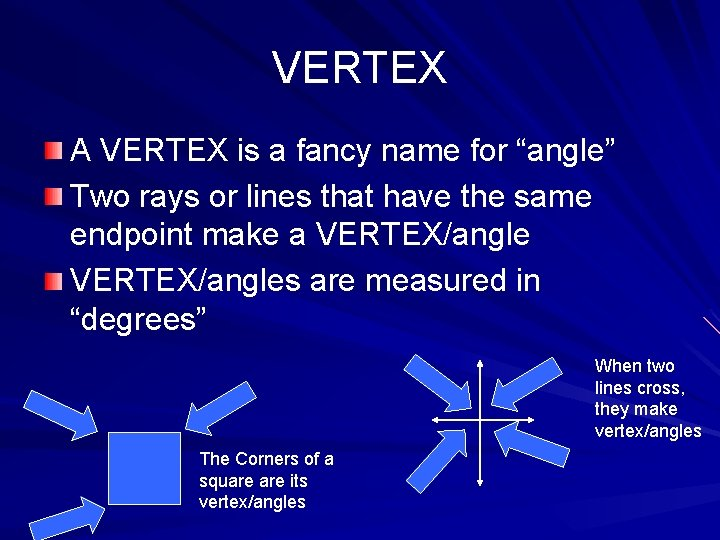VERTEX A VERTEX is a fancy name for “angle” Two rays or lines that have the same endpoint make a VERTEX/angles are measured in “degrees” When two lines cross, they make vertex/angles The Corners of a square its vertex/anglesVERTICAL LINE A VERTICAL LINE goes up & down The candy bars are vertical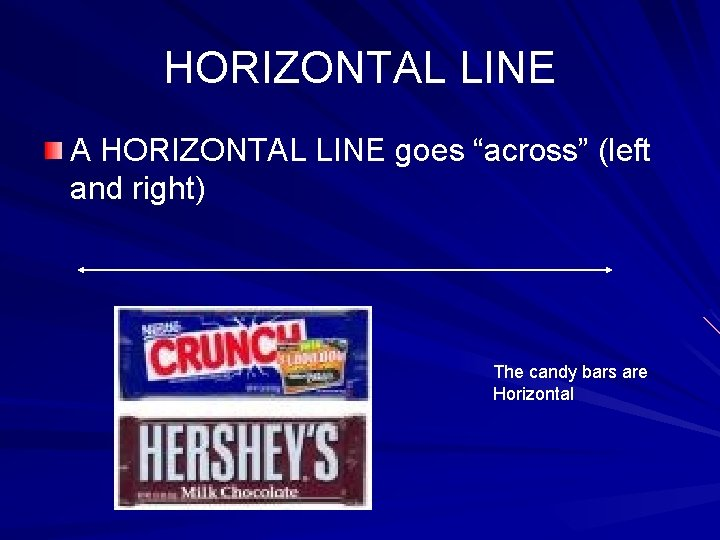HORIZONTAL LINE A HORIZONTAL LINE goes “across” (left and right) The candy bars are Horizontal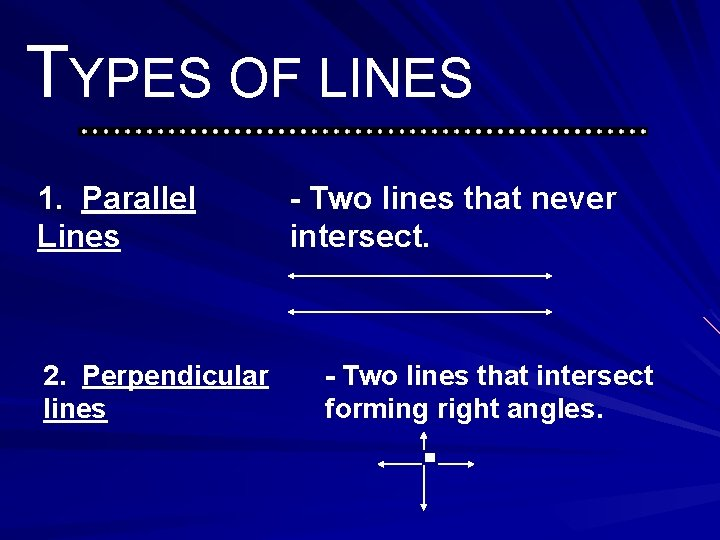TYPES OF LINES 1. Parallel Lines 2. Perpendicular lines - Two lines that never intersect. - Two lines that intersect forming right angles.PLANE § A PLANE (no, not the one that flies!) is a flat surface that goes on forever in all directions. § Imagine sitting on a row boat in the middle of the ocean. No matter which way you look…all you see is water…forever.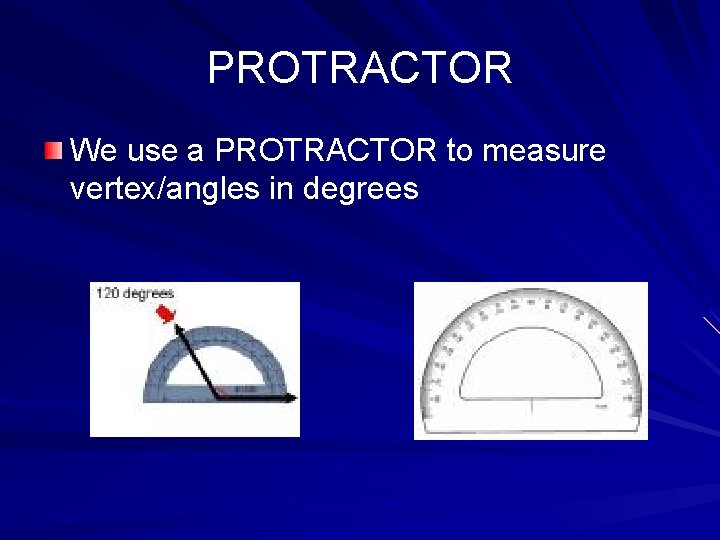PROTRACTOR We use a PROTRACTOR to measure vertex/angles in degrees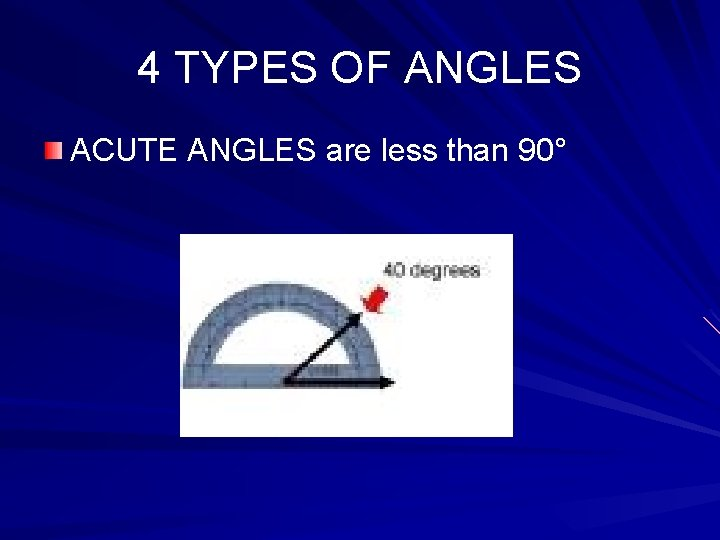4 TYPES OF ANGLES ACUTE ANGLES are less than 90°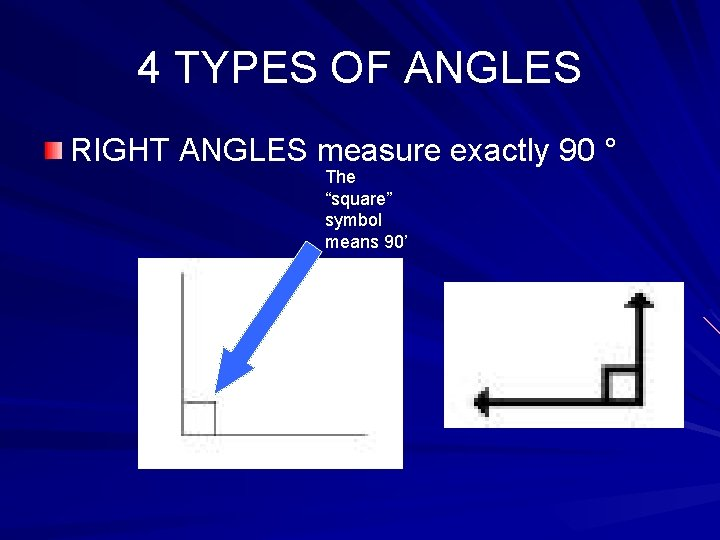4 TYPES OF ANGLES RIGHT ANGLES measure exactly 90 ° The “square” symbol means 90’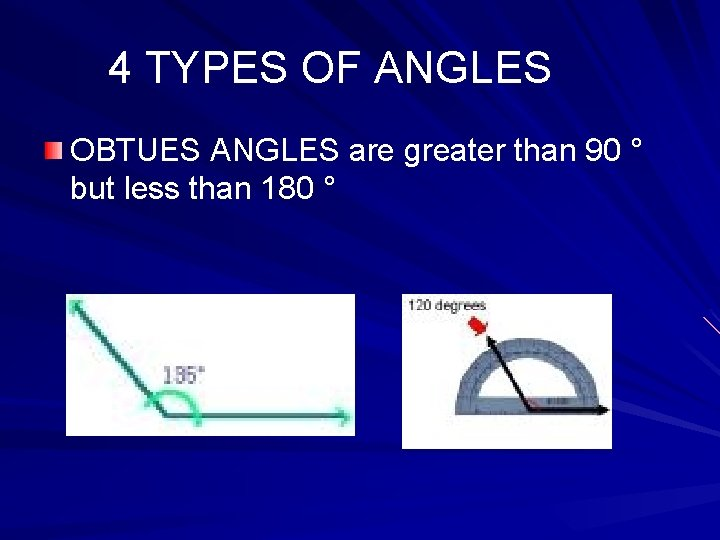4 TYPES OF ANGLES OBTUES ANGLES are greater than 90 ° but less than 180 °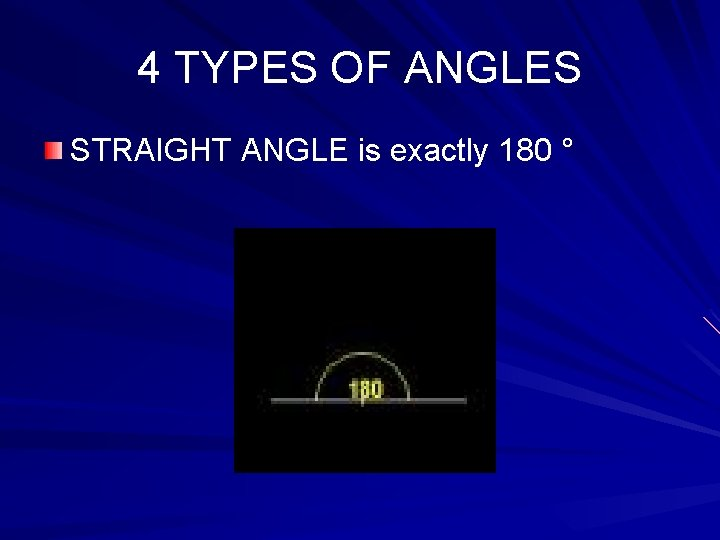4 TYPES OF ANGLES STRAIGHT ANGLE is exactly 180 °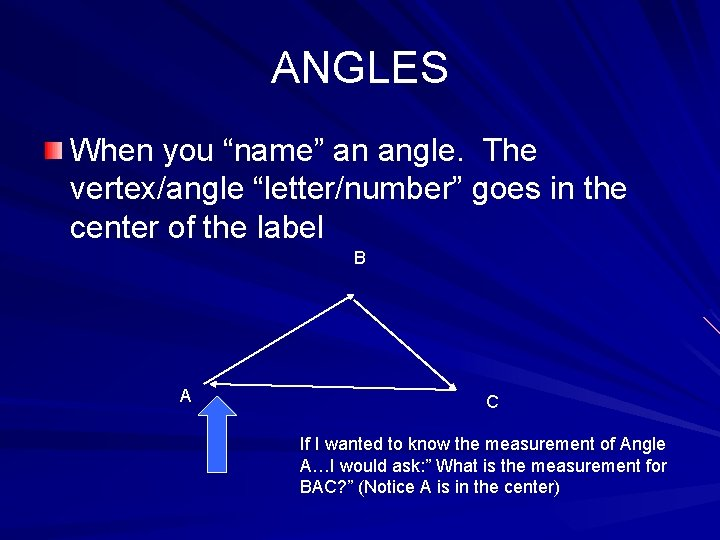ANGLES When you “name” an angle. The vertex/angle “letter/number” goes in the center of the label B A C If I wanted to know the measurement of Angle A…I would ask: ” What is the measurement for BAC? ” (Notice A is in the center)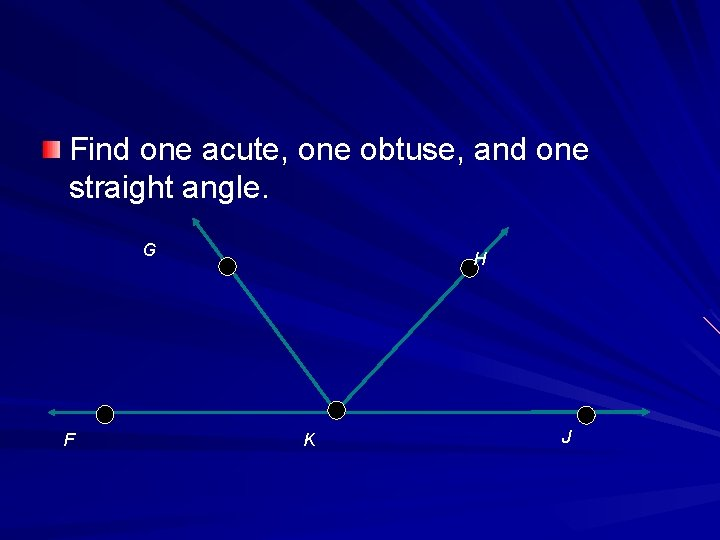Find one acute, one obtuse, and one straight angle. G F H K J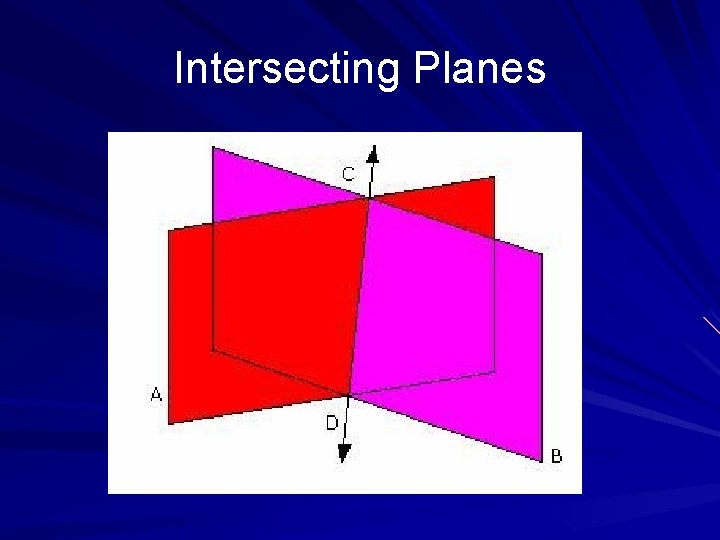Intersecting Planes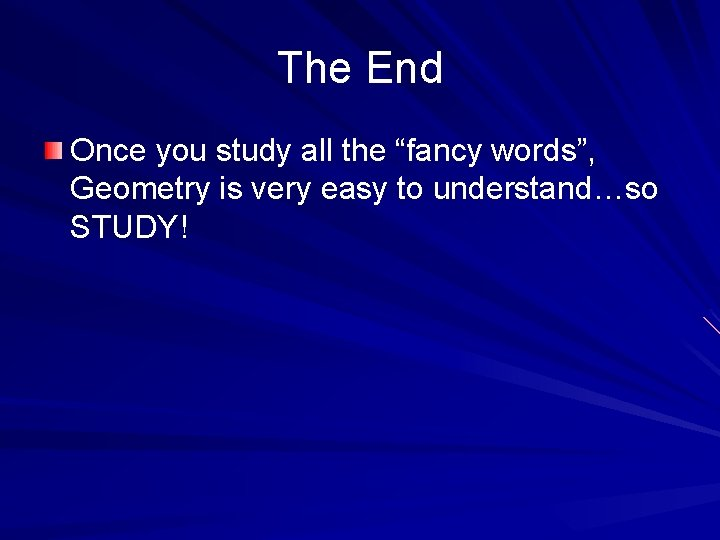The End Once you study all the “fancy words”, Geometry is very easy to understand…so STUDY!